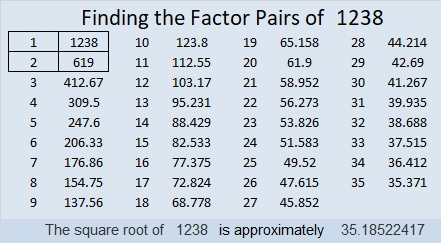# 1238 and Level 6

If you use logic, you can figure out the solution to this puzzle. You will have to study all the clues just to know where to start, but I think you’ll find a lot of satisfaction in finding the solution.Print the puzzles or type the solution in this excel file: 12 factors 1232-1241

Now I’ll share some facts about the number 1238:

• 1238 is a composite number.
• Prime factorization: 1238 = 2 × 619
• The exponents in the prime factorization are 1 and 1. Adding one to each and multiplying we get (1 + 1)(1 + 1) = 2 × 2 = 4. Therefore 1238 has exactly 4 factors.
• Factors of 1238: 1, 2, 619, 1238
• Factor pairs: 1238 = 1 × 1238 or 2 × 619
• 1238 has no square factors that allow its square root to be simplified. √1238 ≈ 35.18522Because of its prime factors, I know that 1238 is part of only one Pythagorean triple:
1238-383160-383162

1238 is a palindrome in two other bases:
It’s 646 in BASE 14, and
it’s 383 in BASE 19.